output.to from Sideway
Draft for Information Only

# Content

`Fluid Motion Linear Translation Linear Deformation Linear Strain Rate Angular Rotation Angular Deformation Angular Strain Rate`

# Fluid Motion

The fluidity property of fluid makes the motion of fluid very complex. The velocity variation of each fluid particle during fluid flow within the velocity fieldcaused by forces results fluid element deformation. The deformation of fluid is similar to that of solid except fluid deforms continuously and therefore express in terms of rate of deformation or strain rate. Types of fluid motion and deformation are translation, linear deformation, rotation, and angular deformation.

## Linear Translation

The linear translation of a fluid element in a small time interval with same field velocity along the flow direction, for example in xy plane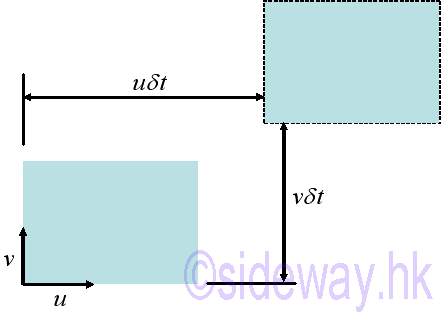## Linear Deformation

The linear deformation of a fluid element in a small time interval with field velocity gradient along the flow direction, for example in xy plane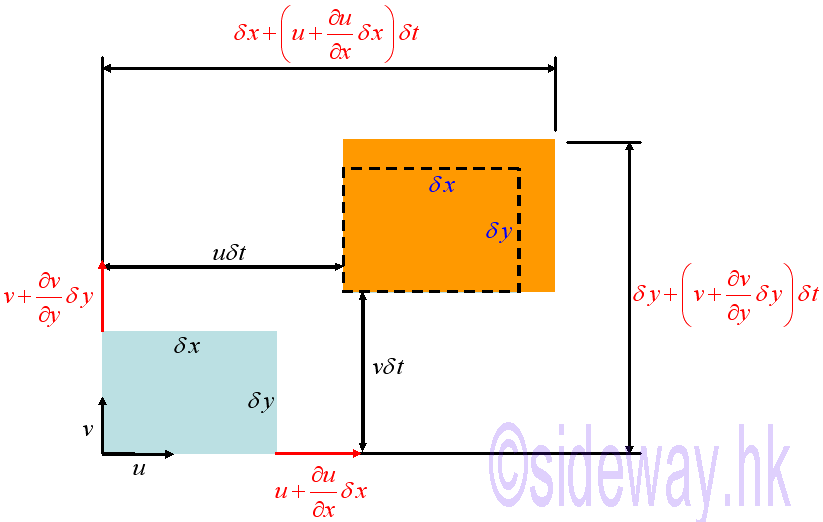## Linear Strain Rate

The linear strain rate or the rate of change of length per unit length along one of the flow direction can be expressed as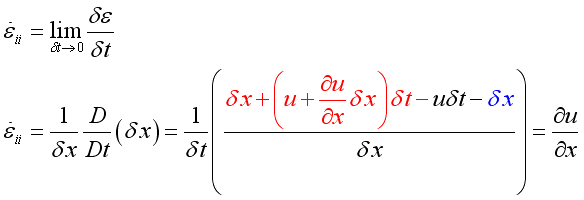Let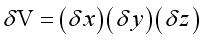, the volumetric strain rate or the bulk strain rate is then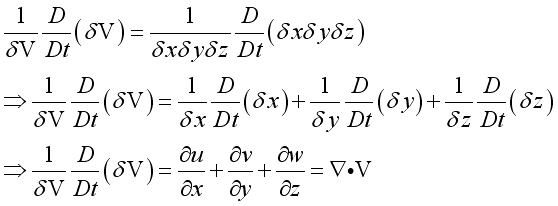## Angular Rotation

When there is a cross flow velocity gradient along the flow, the fluid element will rotate, for example in the xy-plane,## Angular Deformation

In a small time interval, the angular deformations of the fluid element, for example in the xy-plane.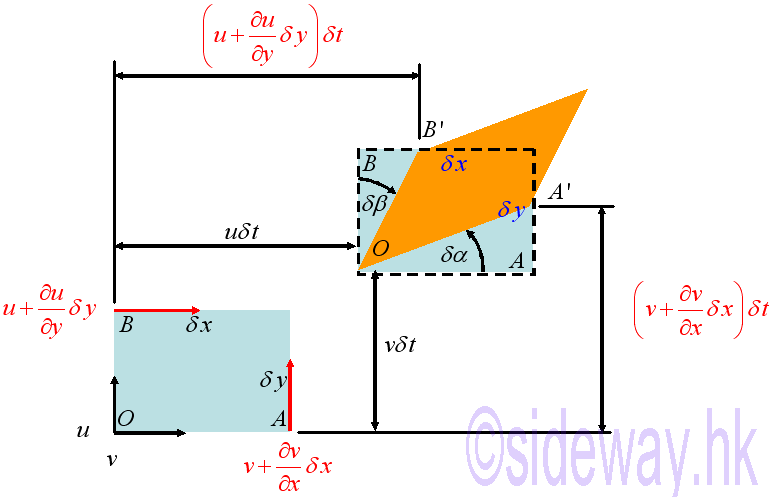Therefore for small angles,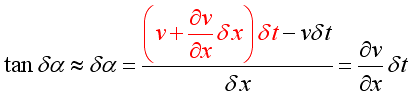and## Angular Strain Rate

The angular strain rate or the rate of shear strain by definition can be expressed asand in terms of strain rate tensor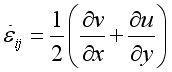ID: 100200003 Last Updated: 2/10/2010 Revision: 1Home 5

Management

HBR 3

Information

Recreation

Culture

Chinese 1097

English 339

Reference 79Computer

Hardware 224Software

Application 213

Latex 52Manim 204

KB 1

Numeric 19

Programming

Web 289

Unicode 504

HTML 66

CSS 65

SVG 46

ASP.NET 270

OS 429

Python 72

Knowledge

Mathematics

Algebra 84

Geometry 33Calculus 67

Engineering

Mechanical

Rigid Bodies

Statics 92

Dynamics 37

Fluid Kinematics 5

Control

Natural Sciences

Electric 27